Free Shipping On All Orders!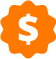Today's Deals

# Glass Weight Calculator

Calculate the weight of your glass and mirror products in a few easy steps!

Customize your glass below with shape, size, thickness, edges, and more. Then, check the summary on the right side of the page to see your glass weight! You can even buy your glass online with free, easy home delivery!

(Weight calculations are approximate.)

### 2. Enter dimensions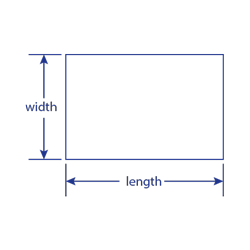Inches Fraction of an Inch
All measurements are in inches
Width:
Length: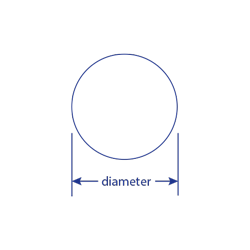Inches Fraction of an Inch
All measurements are in inches
Diameter: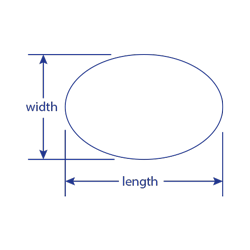Inches Fraction of an Inch
All measurements are in inches
Width:
Length: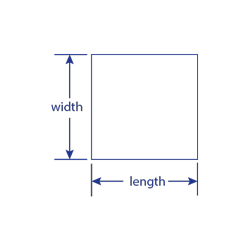Inches Fraction of an Inch
All measurements are in inches
Width / Length:

### 8. Choose Center Hole

Figures are approximate — Dulles Glass and Mirror does not guarantee accuracy and is not responsible for any miscalculations.

### Summary

Glass Weight:
• List UPrice
• Discount
• Online UPrice
• Ships within 1-3 business days.
• ##### Accessories

How do I figure glass weight per square foot of glass?

Multiply 1.7 pounds for every 1/8" square foot of glass. For example, a 3/8" panel of glass that is 3 x 6 would be 1.7 x 3 (from 3/8") x 3 x 6 = 91.8 pounds.

Here are common, approximate weights for glass per square feet:

• SS - 1.20
• DS - 1.60
• 5/32 - 2.00
• 3/16 - 2.45
• 1/4 - 3.00
• 3/8 - 5.00
• 0-1/2 - 6.50
• 5.8 - 8.00
• 3/4 - 9.97
• IG unit - 2 x glass
How do I figure the square footage of the glass I need?

For square and rectangular glass, square footage is determined by multiplying the width x the length of the table and dividing the answer by 144. You will need two measurements of the glass. Use the tape measure to measure the length and the width of the glass in inches. Next, multiply the two measurements of the glass. Divide the answer by 144. This will give you the square footage of the glass.

To determine the square footage of a round glass, measure the diameter of the glass using the tape measure (the diameter is the length across the glass directly through the center). Divide the diameter in half. The resulting number is the "radius". Convert the radius measurement to decimal by dividing the inches portion of the measurement by 12 (for example, a glass with a radius of 3 feet 9 inches has a decimal radius of 3.75 feet).

Now, calculate the area of the glass using the formula for the area of a circle: Area equals pi times the radius squared. Pi is a constant value of 3.1416. To square is to multiply by the number by itself. In the example, the round glass calculation will be 3.1416 x 3.75 x 3.75 equals 44.18 square feet, rounded to two places.

The area of an oval glass can be calculated by thinking of the glass as a rectangle with half of a round glass on each end. Do the calculations for the rectangle and round parts and add them together.

What are the decimal equivalents to the glass size in fractions?

Here are the decimal vs. fraction equivalents:

• 1/16 - .0625
• 1/8 - .125
• 3/16 - .1875
• 1/4 - .250
• 5/16 - .3125
• 3/8 - .375
• 7/16 - .4375
• 1/2 - .500
• 9/16 - .5625
• 5/8 - .625
• 11/16 - .6875
• 3/4 - .750
• 13/16 - .8125
• 7/8 - .875
• 15/16 - .9375
• 1 â€“ 1.000